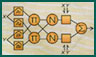## Addenda of Neuro-Fuzzy and Soft Computing

### by J.-S. R. Jang, C.-T. Sun, and E. Mizutani

1. Addendum to Section 5.2, "Basics of Matrix Manipulation and Calculus":
List of handy formulas for gradients and Jacobian
2. Addendum to Section 5.2, "Basics of Matrix Manipulation and Calculus":
Formulas for matrix inversion in block form
3. Addendum to Section 5.5, "Recursive Least-Squares Estimator":
Incremental formula for LSE error measures
4. Addendum to Section 5.5, "Recursive Least-Squares Estimator":
Recursive LSE in the number of parameters
5. Addendum to Section 5.8, "LSE for Nonlinear Models":
List of nonlinear models that can be transformed into linear ones
The Book's Home Page, or Roger's Home Page